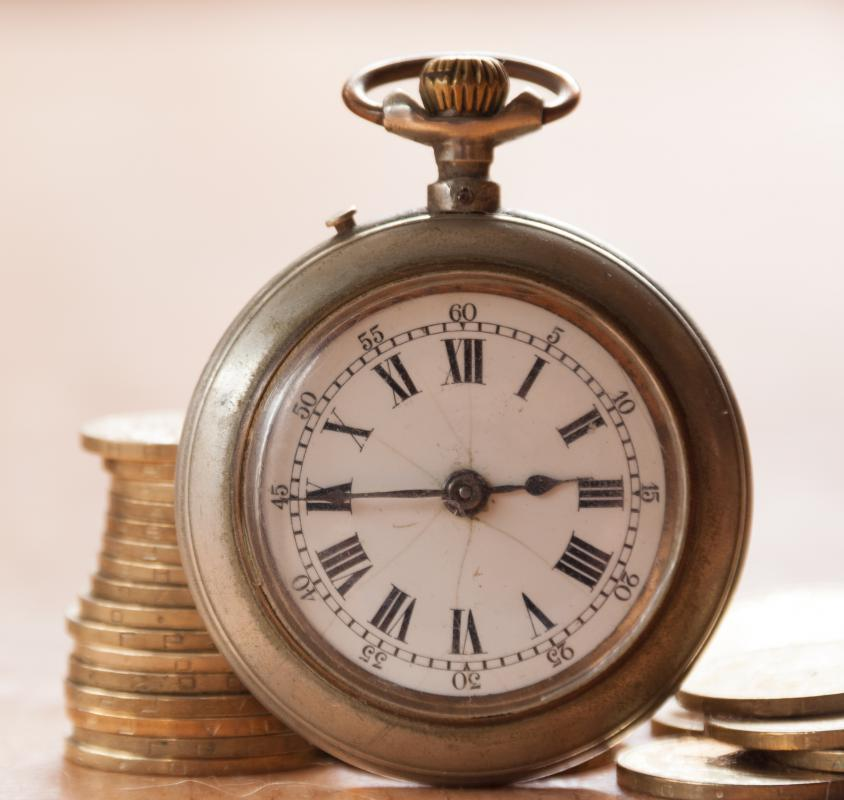# What is the Velocity of Money?

Leo Zimmermann

The velocity of money is the speed with which money moves through an economy. It is calculated by dividing the value of all the economy's transactions by the total amount of money available. The result is a number that describes how many times any given chunk of money was spent over a period of time—usually one year.The velocity of money is the speed at which it moves through an economy.

As an example, consider a small economy made up of three authors who continually sell books to each other using wampum beads as currency. If each starts out with 20 wampum beads, the total amount of money available in in the economy is 60 beads. This number is also called the money supply. If each of these prolific authors buys a book from both of the others for 10 beads each, each individual still has 20 beads, but 60 total beads worth of books have been purchased. If they repeat this behavior every three-month season, the economy will have sustained 240 beads worth of book purchases over the course of a year. This number is equivalent to the gross domestic product (GDP) of a national economy.

The velocity of money in the example economy is calculated by dividing the gross domestic product by the money supply. Divide 240 beads per year by 60 beads, and you get a result of '4 per year.' Note that the final result does not contain units of currency; it could refer to individual beads, to strings of five or ten beads, or even to some observer's estimate of the worth of beads in dollars. The only unit is the space of time in which the transactions are taking place. This number means that an average chunk of currency changes hands four times in the course of a year.

The same simple calculation can be performed to determine the 2008 velocity of money in the United States. According to the CIA Factbook, the United States had a GDP of \$14.61 trillion US Dollars (USD) and a money supply of \$1.436 trillion USD. The velocity of money was therefore 10.17. That is, the average dollar within the economy changed hands approximately ten times. Of course, this final number is a very rough estimate, since in practice it is difficult to account for every transaction that occurs. The black market alone represents a large source of rapid currency turnover not represented in the official GDP.

The velocity of money is still considered an important calculation by economists, because it may help predict economic outcomes. All else being equal, a high velocity of money is considered good because it suggests the existence of a healthy and productive economy. However, high velocity of money can also suggest the existence of inflation, since money circulates faster when people have to use more of it in any given transaction.

## You might also Like